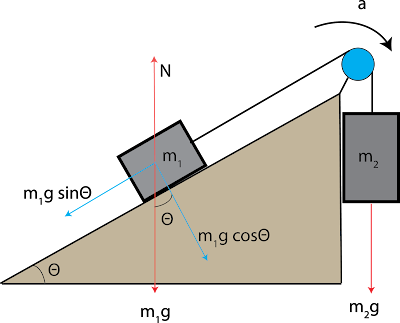21 May 2017

How to solve the pulley problems (on a inclined plane)
Pulleys on a flat surface
Let's talk about a different type of problem with using pulleysPulley on an inclined plane
How to solve pulley on incline plane$$a=\dfrac{m_2g-m_1g sin\theta}{m_1+m_2}$$

$$T_1=m_1g\pm m_1a$$ Going up
$$T_1=m_1g+ m_1a$$

$$T_2=m_2g\pm m_2a$$ Going down
$$T_2=m_2g- m_2a$$

Now what if we consider friction now?

Now tilt it a little bit.$$\dfrac{m_2g sin\theta_2-m_1g sin\theta_1}{m_1+m_2}$$
$$T_1=T_2$$
$$T_2=m_2g sin\theta_2\pm m_2a$$
$$T_2=m_2g sin\theta_2- m_2a$$

See also: Pulley Hanging from the celling

Physics Graduate and Data Science Enthusiast.